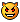vect a kindof better array ;-)

Recommended Posts

Why ?Because u can't have an empty array !So I start a vector kindof implementation.

Where u can have an empty vectorReally useful !Nota, the first element is allways the size of the vector .

#include-once

func vectNew( \$a1 = 0, \$a2 = 0, \$a3 = 0, \$a4 = 0, \$a5 = 0, \$a6 = 0, \$a7 = 0, \$a8 = 0, \$a9 = 0, \$a10 = 0 )
local \$p = @NumParams
local \$nv
local \$i

if \$p > 0 then
redim \$nv[\$p + 1]
for \$i = 1 to \$p
\$nv[\$i] = Eval( "a" & string( \$i ) )
next
\$nv = \$p
else
\$nv = 0
endif
return( \$nv )
endfunc

func vectAdd( byref \$v, \$a1 = 0, \$a2 = 0, \$a3 = 0, \$a4 = 0, \$a5 = 0, \$a6 = 0, \$a7 = 0, \$a8 = 0, \$a9 = 0, \$a10 = 0 )
local \$i, \$vns
local \$p = @NumParams

if \$p > 1 then
\$vns = \$v + \$p
redim \$v[\$vns]
for \$i = 1 to \$p - 1
\$v[\$v + \$i] = Eval( "a" & string( \$i ) )
next
\$v = \$vns - 1
endif
return( \$v )
endfunc

func vectInclude( byref \$v, \$val )
local \$i

for \$i = 1 to \$v
if \$v[\$i] = \$val then
return( \$i )
endif
next
return( -1 )
endfunc

func vectRemoveIndex( byref \$v, \$index )
local \$i

if \$index > 0 and \$index <= \$v then
for \$i = \$index to \$v - 1
\$v[\$i] = \$v[\$i + 1]
next
redim \$v[\$v]
\$v -= 1
endif
return( \$v )
endfunc

func vectDisplay( byref \$v, \$title )
local \$i
local \$str = ""

for \$i = 0 To ubound( \$v ) - 1
\$str &= "[" & \$i & "] = " & stringStripCR( \$v[\$i]  ) & @CR
next
msgbox( 4096, \$title, \$str )
endfunc

global \$v = vectNew()
global \$i

vectDisplay( \$v, "v" )
vectAdd( \$v, 1, 3, 4, 2 )
vectDisplay( \$v, "v" )

\$i = vectInclude( \$v, 3 )
vectRemoveIndex( \$v, \$i )
vectDisplay( \$v, "v" )
Edited by JoeCool
Share on other sites

Yes this is a great idea you are using. What you call a Vector is what is also called a 1-based array (since the first element is in the 1 index instead of the 0 index). All the routines I write use 1-based arrays, because you don't have to ReDim them all the time. My Array1Add() routine looks something like this:

\$aSource = \$aSource + 1
If \$aSource >= UBound(\$aSource) Then
ReDim \$aSource[\$aSource * 2]
EndIf
\$aSource[\$aSource] = \$vValue
EndFunc

I call my functions Array1* because they deal with 1-based arrays.

Share on other sites

\$aSource = \$aSource + 1
If \$aSource >= UBound(\$aSource) Then
ReDim \$aSource[\$aSource * 2]
EndIf
\$aSource[\$aSource] = \$vValue
EndFunc

I call my functions Array1* because they deal with 1-based arrays.

<{POST_SNAPBACK}>

Just a remark on your code, if u want to resize your array one in a while, use somethink like

ReDim \$aSource[\$aSource + 20 ] instead of ReDim \$aSource[\$aSource * 2]

so you while not "overgrow" your array for nothing when you get a big array

(1000 elements or more ... )

your array size now are 1, 2, 4, 8, 16, ..., 512, 1024, 2048 etc ...

of course you can also shrink you array in the same way

\$aSource[\$aSource - 20 ]

;-)

Share on other sites

Fabulous.. Very nice code. You might want to document your functions so that their usage is much more clear.

Cheers..Share on other sites

Just a remark on your code,  if u want to resize your array one in a while, use somethink like

ReDim \$aSource[\$aSource + 20 ]  instead of    ReDim \$aSource[\$aSource * 2]

so you while not "overgrow" your array  for nothing when you get a big array

(1000 elements or more  ... )

your array size now are 1, 2, 4, 8, 16, ..., 512, 1024, 2048  etc ...

of course you can also shrink you array  in the same way

\$aSource[\$aSource - 20 ]

;-)

<{POST_SNAPBACK}>

I go back and forth on that issue. Usually I end up having to customize for the use I need. Sometimes I want an array to grow steadily (by adding 20 or 50 or so) and sometimes I just want to make sure that I do as few increases as possible. I usaully start my array out with 100 elements (Dim \$aMyArray, and \$aMyArray evaluates to zero, so the first element will always be set to ) I figure that if I fill up 100 elements, it's not unreasonable to assume that I might fill up 100 more. And if I fill those 200, then it's not unreasonable to think that I might fill up 200 more, etc. So starting with 100 and doubling from there has been a good basis to start with. And like I said, I customize it if I need to.

And for trimming the array - well, if you know you're done adding, then ReDim \$aMyArray[\$aMyArray + 1] works pretty well.To see some examples of how I use 1-based arrays, see my Table and Binary Tree UDFs:

http://www.autoitscript.com/forum/index.php?showtopic=13114

http://www.autoitscript.com/forum/index.php?showtopic=12136

Edited by blindwig

Create an account

Register a new account